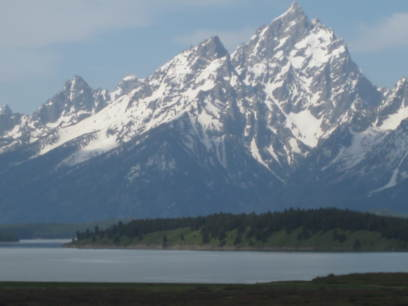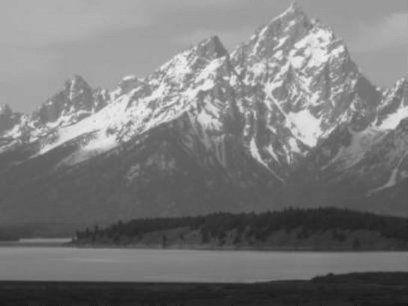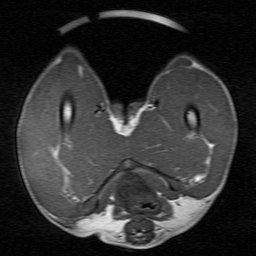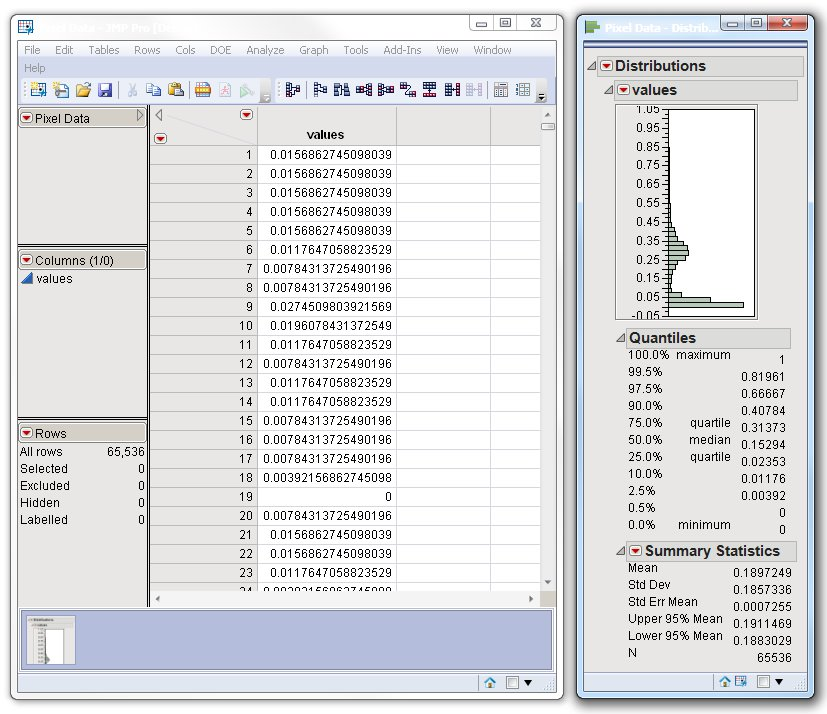Our World Statistics Day conversations have been a great reminder of how much statistics can inform our lives. Do you have an example of how statistics has made a difference in your life? Share your story with the Community!
Choose Language Hide Translation Bar
Improve your image with JMP 9

If you attended the JMP Discovery Summit 2011 in Denver, Colorado, back in September, you may have sat in on my presentation, Image Isn't Everything But It Sure Is Something in JMP 9. I talked about the image functionality that was added to JMP for version 9, and I demonstrated some real-life examples of how JMP users are utilizing the image functionality to extract data contained in their images. I shared some JMP Scripting Language (JSL) scripts in hopes that it might jump-start (pun-intended) efforts to use the image functionality. For that same reason, I decided to put together a JSL file of code snippets that also demonstrates the image functionality. That file, called ImageExamples.jsl, can be found in the JMP File Exchange. Let's pull a few examples out of that file and talk a little more about them.

The first thing you might want to do is get an existing image into JMP. To do this, simply use the New Image command.

img = newImage( "C:\Users\sasjvp\Pictures\Yellowstone08\IMG_0262.JPG" );

newWindow( "Original Image", img );This example reads in a JPEG image and stores it in JSL as an image object, called img. NewImage() can read JPG, PNG, BMP, GIF and TIF files.

When I first did this, the resultant window was very large, due to the fact that the image was so large. I wondered what the dimensions of the image actually were, so I did this:

{w, h} = img << getSize();

This command gave me the width and height of the image, which was 3264 x 2448, a rather large image. So to display it in a more reasonable size, I scaled it down by specifying a new width and height for the image.

img << setSize( {w/4, h/4} );

Now the image is 816 x 612, or one-fourth the original size in each dimension. Notice that the width and height are passed as a list, by wrapping them in curly brackets.

Now I want to manipulate the image, but I want to preserve the original version. So I will create a copy of my image by using NewImage().

img2 = newImage( img );

This creates a copy of my original image as a second image, called img2.

The next thing I want to do is get the pixel values of the image. Since my image is a color image, each pixel is defined in terms of three color component:, red, green and blue. The pixel data is returned as a matrix for each color component that I ask for.

{r, g, b} = img2 << getPixels( "rgb" );

In this case, I will get three matrices. If my image had transparency in it, I could ask for four matrices by specifying "rgba". I can also ask for just one color component simply by specifying the component I want, such as "g" for the green component. Each matrix represents the pixel color values in normalized color space, meaning that the values are between 0.0 and 1.0 inclusive.

The nice thing about getting the pixel values as matrices is that I can now do matrix math on my pixels. If I wanted to create a gray-scale version of my image, I could do this very simply with the following formula.

i = .30*r + .59*g + .11*b;

This is the same formula that a TV would use to convert a color show into black and white. If you are as old as me, you might remember black-and-white television, which wasn't just black and white, but rather it included a range of gray between black and white. This formula combines 30% of my red component with 59% of my green component and 11% of my blue component. This calculates an intensity value for my gray scale. Now I can replace the original color components of red, green and blue with the gray scale intensity values to produce a gray-scale image.

img2 << setPixels( "rgb", {i, i, i} );

Here is the result.Since JMP supports matrix math in JSL, we don't have to traverse the image and manipulate each pixel individually. We can apply this formula to the entire image at one time, which is both convenient and efficient.Of course, the real strength of JMP is its ability to do statistical analysis and data discovery. To do this, we need the data to be in the form of a data table. So let's read in an image, extract the pixel data and create a data table from it.

Let's look at an image taken from an MRI. We can use the NewImage() command as before to read the image into JMP. We can also use the getPixels() command as before except that this image is a gray-scale image already, so we only need to ask for one color component. The first component is still referred to as the "r" component. Once we have the pixel values we can create the data table.

img = NewImage("C:\JMP\mri.jpg");

{pix} = img << getPixels("r");

dt = New Table("Pixel Data",

newColumn("values", set values(pix)));

Distribution( Continuous Distribution( Column( :values ), Outlier Box

Plot( 0 ) ) );

new window("Analyze Image Data", img);

The pixel values are now in a data table just like any other data read into JMP. This means we can now use the statistical analysis features in JMP to analyze the data. What to use at this point depends on what your data is and what questions you are trying to answer. In this example, I did a simple Distribution to show me what the range and frequency of my data is.I hope that by this point I've given you enough examples to get you started using images in JMP. Don't forget to download the examples script, ImageExamples.jsl, from the JMP File Exchange. It shows more examples than I've mentioned here. It includes commands for rotation, transparency and filters, among other things. Let me know what you think.

Article Labels

There are no labels assigned to this post.

1 Comment
Visitor

Image manipulation in JMP using JSL wrote:

[...] The command, getPixels("rgb"), returns each image as a collection of three matrices -- one for each color component, red, green and blue. To blend the images, we multiply Image2 by the percent and then add that to Image1 multiplied by 1 minus the percent. We then set that as the resultant image, Image3. That's all there is to it. For a further explanation of getPixels(), as well as other image functionality, check out another blog post of mine, Improve your image with JMP 9. [...]